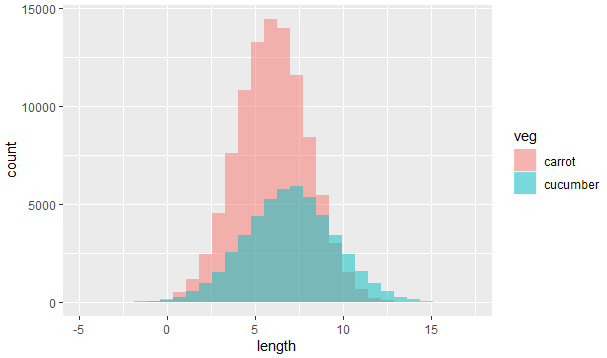2 views

I am using R and I have two data frames: carrots and cucumbers. Each data frame has a single numeric column which lists the length of all measured carrots (total: 100k carrots) and cucumbers (total: 50k cucumbers).

I wish to plot two histogram - carrot length and cucumbers lengths - on the same plot. They overlap, so I guess I also need some transparency. I also need to use relative frequencies not absolute numbers since the number of instances in each group is different.

something like this would be nice but I don't understand how to create it from my two tables:closed

by
selected

To plot two histograms together in R, follow these steps:

Combine the data frames:

carrots <- data.frame(length = rnorm(100000, 6, 2))

cucumbers <- data.frame(length = rnorm(50000, 7, 2.5))

carrots\$veg <- 'carrot'

cucumbers\$veg <- 'cucumber'

Lengths <- rbind(carrots, cucumbers)

Plot the data:

ggplot(Lengths, aes(length, fill = veg)) +

geom_histogram(alpha = 0.5, position = 'identity')

Output: